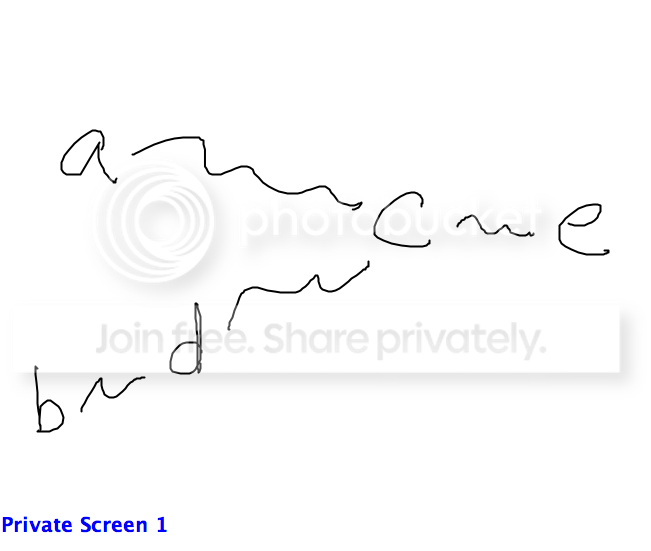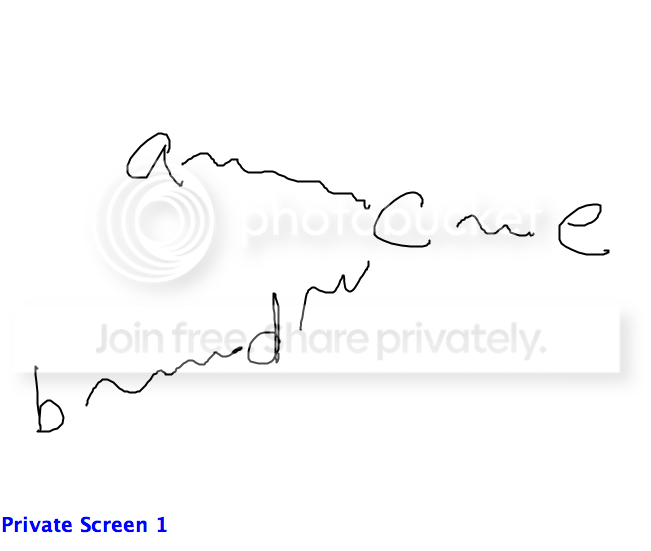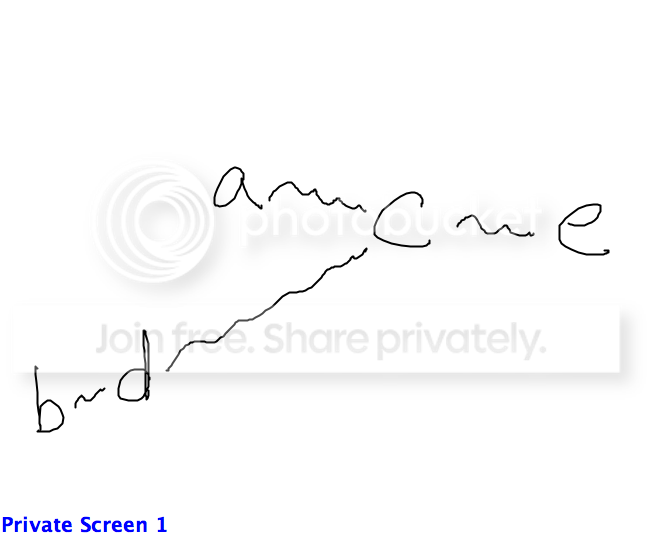GMAT Question of the Day: Daily via email | Daily via Instagram New to GMAT Club? Watch this Video

 It is currently 19 Feb 2020, 20:07### GMAT Club Daily Prep

#### Thank you for using the timer - this advanced tool can estimate your performance and suggest more practice questions. We have subscribed you to Daily Prep Questions via email.

Customized
for You

we will pick new questions that match your level based on your Timer History

Track

every week, we’ll send you an estimated GMAT score based on your performance

Practice
Pays

we will pick new questions that match your level based on your Timer History

#### Not interested in getting valuable practice questions and articles delivered to your email? No problem, unsubscribe here.# Is the median of the five numbers a,b,c,d,e and e equal to d?

Author Message
TAGS:

### Hide Tags

DirectorB
Status: I don't stop when I'm Tired,I stop when I'm done
Joined: 11 May 2014
Posts: 518
GPA: 2.81
Is the median of the five numbers a,b,c,d,e and e equal to d?  [#permalink]

### Show Tags

1700:00

Difficulty:25% (medium)

Question Stats:75% (01:29) correct25% (01:18) wrongbased on 892 sessions

### HideShow timer Statistics

Is the median of the five numbers a,b,c,d and e equal to d?

(1) a<c<e
(2) b<d<c

OG Q 2017 New Question
DirectorB
Joined: 04 Jun 2016
Posts: 537
GMAT 1: 750 Q49 V43
Re: Is the median of the five numbers a,b,c,d,e and e equal to d?  [#permalink]

### Show Tags

1
AbdurRakib wrote:
Is the median of the five numbers a,b,c,d and e equal to d?

(1) a<c<e
(2) b<d<c

OG Q 2017 New Question

E

Statement 1) Insufficient doesn't tell about b and d (Option A and D out)
Statement 2) Insufficient doesn't tell about a or e (Option B out)

Merge both statement 1 & 2) :- Still Insufficient
All we know a,b, and d are < c we do not know the exact magnitude so d can be the first , second or third element of the set in ascending value. (Option C out)

HENCE E
_________________
Posting an answer without an explanation is "GOD COMPLEX". The world doesn't need any more gods. Please explain you answers properly.
FINAL GOODBYE :- 17th SEPTEMBER 2016. .. 16 March 2017 - I am back but for all purposes please consider me semi-retired.
Senior ManagerJoined: 02 Mar 2012
Posts: 263
Schools: Schulich '16
Re: Is the median of the five numbers a,b,c,d,e and e equal to d?  [#permalink]

### Show Tags

E

analyse bothe the equations and there is discrepancy between 'a' and 'd'.

either of them ca be left or right between 'b' and 'c'
DirectorG
Joined: 04 Aug 2010
Posts: 532
Schools: Dartmouth College
Re: Is the median of the five numbers a,b,c,d,e and e equal to d?  [#permalink]

### Show Tags

1
1
AbdurRakib wrote:
Is the median of the five numbers a,b,c,d and e equal to d?

(1) a<c<e
(2) b<d<c

OG Q 2017 New Question

Statements combined:
To combine the two inequalities visually, we can create the following diagram:In the figure above, the squiggly lines are like elastic bands that can stretch or contract.

Case 1:Here, the ordering from left to right is as follows:
b < a < d < c < e
In this case, the median is d.

Case 2:Here, the ordering from left to right is as follows:
b < d < a < c < e
In this case, the median is a.

Since the median can be different values, the two statements combined are INSUFFICIENT.

_________________
GMAT and GRE Tutor
New York, NY

Available for tutoring in NYC and long-distance.
InternB
Joined: 09 May 2017
Posts: 24
Location: India
GMAT 1: 610 Q42 V33GPA: 4
WE: Engineering (Energy and Utilities)
Re: Is the median of the five numbers a,b,c,d,e and e equal to d?  [#permalink]

### Show Tags

AbdurRakib wrote:
Is the median of the five numbers a,b,c,d and e equal to d?

(1) a<c<e
(2) b<d<c

OG Q 2017 New Question

St1 : a<c<e no info about b & d hence NS
St2 : b<d<c no info about a & e hence NS

choice A,B,D can be eliminated
Combining St1 & 2 : NS counter example can be as following:
b<d<a<c<e ( d is not median)

DirectorG
Joined: 19 Oct 2013
Posts: 507
Location: Kuwait
GPA: 3.2
WE: Engineering (Real Estate)
Re: Is the median of the five numbers a,b,c,d,e and e equal to d?  [#permalink]

### Show Tags

AbdurRakib wrote:
Is the median of the five numbers a,b,c,d and e equal to d?

(1) a<c<e
(2) b<d<c

OG Q 2017 New Question

From statement 1) a < c < e

it could be a + b + d + c + e, here d is the median.

it could also be a + d + b + c + e here b is the median. insufficient.

Eliminate A/D

Check statement 2) b < d < c

b + d + c + a + e here c is the median

it could be a + b + d + c + e here d is the median.

insufficient.

now try combining (1) and (2)

it could be a + b + d + c + e here d is the median.

or it could be b + d + a + c + e here a is the median.

Insufficient.

Non-Human UserJoined: 09 Sep 2013
Posts: 14100
Re: Is the median of the five numbers a,b,c,d,e and e equal to d?  [#permalink]

### Show Tags

Hello from the GMAT Club BumpBot!

Thanks to another GMAT Club member, I have just discovered this valuable topic, yet it had no discussion for over a year. I am now bumping it up - doing my job. I think you may find it valuable (esp those replies with Kudos).

Want to see all other topics I dig out? Follow me (click follow button on profile). You will receive a summary of all topics I bump in your profile area as well as via email.
_________________Re: Is the median of the five numbers a,b,c,d,e and e equal to d?   [#permalink] 24 Jan 2020, 06:49
Display posts from previous: Sort by

# Is the median of the five numbers a,b,c,d,e and e equal to d?